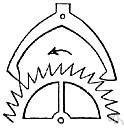(redirected from Ideal mechanical advantage)
Also found in: Thesaurus, Acronyms, Encyclopedia.
Related to Ideal mechanical advantage: Actual mechanical advantage

n.
The ratio of the output force produced by a machine to the applied input force.

n
(Mechanical Engineering) the ratio of the working force exerted by a mechanism to the applied effort

 Noun 1mechanical advantage - the ratio of the force exerted by a machine to the force applied to itratio - the relative magnitudes of two quantities (usually expressed as a quotient)